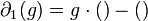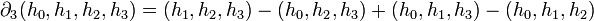# Bar resolution

## Definition

Suppose$G$ is a group. The bar resolution of$G$ is a long exact sequence of$\mathbb{Z}(G)$-modules:$\dots \mathcal{B}_n(G) \to \mathcal{B}_{n-1}(G) \to \dots \mathcal{B}_1(G) \to \mathcal{B}_0(G) \to \mathbb{Z} \to 0$

defined by the following information.

We denote the identity element of$G$ by$1$.

### The groups

The group$\mathcal{B}_n(G)$ is defined as the free abelian group on the set$G^{n+1}$, with$G$ acting on it diagonally:$\! g \cdot (h_0, h_1, h_2, \dots, h_n) = (gh_0, gh_1, gh_2, \dots, gh_n)$

This group can thus be regarded as a$\mathbb{Z}(G)$-module.

As a$\mathbb{Z}G$-module,$\mathcal{B}_n(G)$ has a free generating set identified by$G^n$ by:$\! (g_1 \mid g_2 \mid \dots \mid g_n) \leftrightarrow (1,g_1,g_1g_2,g_1g_2g_3,\dots,g_1g_2g_3\dots g_n)$

The notation with bars$(\ \mid \ \mid \dots \mid \ )$ is termed the bar notation.

### The derivation in the original notation

The derivation$\partial_n$ in the original notation is given by:$\! \partial_n(h_0, h_2, \dots, h_{n-1},h_n) = \sum_{i=0}^n (-1)^i (h_0, h_1, \dots, \hat{h_i}, \dots h_n)$

### The derivation with the bar notation

The map$\! \partial_n: \mathcal{B}_n(G) \to \mathcal{B}_{n-1}(G)$ is defined as follows:$\! \partial_n(g_1 \mid g_2 \mid \dots \mid g_n) = g_1 \cdot (g_2 \mid g_3 \mid \dots \mid g_n) - (g_1g_2 \mid g_3 \mid \dots \mid g_n) + (g_1 \mid g_2g_3 \mid \dots \mid g_n) - \dots + (-1)^{n-1}(g_1 \mid g_2 \mid \dots \mid g_{n-1}g_n) + (-1)^n(g_1 \mid g_2 \mid \dots \mid g_{n-1})$

In the more precise summation notation:$\! \partial_n(g_1 \mid g_2 \mid \dots \mid g_n) = g_1 \cdot (g_2 \mid g_3 \mid \dots \mid g_n) + \left[\sum_{i=1}^{n-1} (-1)^i (g_1 \mid g_2 \mid \dots \mid g_{i-1} \mid g_ig_{i+1} \mid \dots \mid g_n) \right] - (g_1 \mid g_2 \mid \dots \mid g_{n-1})$

## Particular cases

###$\! \partial_1$

In the comma notation, we have:$\! \partial_1(h_0, h_1) = (h_1) - (h_0)$

The source of$\partial_1$ is$\mathcal{B}_1(G)$, which is a free$\mathbb{Z}(G)$ module on$(g)_{g \in G}$. The target of$\partial_1$ is$\mathcal{B}_0(G)$, which is simply$\mathbb{Z}G$.$\! \partial_1(g) = g \cdot () - ()$

###$\! \partial_2$

In the comma notation, we have:$\! \partial_2(h_0, h_1,h_2) = (h_1,h_2) - (h_0,h_2) + (h_0,h_1)$

The source of$\partial_2$ is$\mathcal{B}_2(G)$, which is a free$\mathbb{Z}(G)$-module on$G \times G$. The target of$\partial_2$ is$\mathcal{B}_1(G)$, which is the free$\mathbb{Z}G$-module on$G$.$\! \partial_2(g_1 \mid g_2) = g_1 \cdot (g_2) - (g_1g_2) + (g_1)$

###$\! \partial_3$

In the comma notation, we have:$\! \partial_3(h_0, h_1,h_2,h_3) = (h_1,h_2,h_3) - (h_0,h_2,h_3) + (h_0,h_1,h_3) - (h_0,h_1,h_2)$

In the bar notation, we have:$\! \partial_3(g_1 \mid g_2 \mid g_3) = g_1 \cdot (g_2 \mid g_3) - (g_1g_2 \mid g_3) + (g_1 \mid g_2g_3) - (g_1 \mid g_2)$